# AP Chemistry : Thermochemistry and Kinetics

## Example Questions

### Example Question #4 : Reaction Energy Profile

Which of the following is true regarding activation energy?

I. Activation energy depends on the pressure of the system

II. The reaction rate decreases as the activation energy increases

III. Catalysts increase the activation energy of a reaction

II only

I only

I and III

I and II

II only

Explanation:

Activation energy is the energy barrier that needs to be overcome for a reaction to proceed; the higher the activation energy, the slower the reaction. Activation energy can only be altered via a catalyst. Catalysts are chemical substances that lower the activation energy, allowing reactions to proceed faster. Other physical quantities such as temperature and pressure don’t alter the activation energy.

Reaction rate is a direct measure of the speed of a reaction. As mentioned, increasing activation energy will increase the barrier and, therefore, slow down the reaction.

Catalysts are chemical substances that decrease the activation energy, thereby increasing the reaction rate. They are commonly used in chemical reactions to drastically speed up reactions that might otherwise take hours or days to complete. Enzymes are biological catalysts that facilitate most of the biological reactions happening in our bodies.

### Example Question #5 : Reaction Energy Profile

__________ the activation energy of a reaction will __________ the amount of products produced.

Increasing . . . increase

Decreasing . . . decrease

Increasing . . . not change

Decreasing . . . increase

Increasing . . . not change

Explanation:

This question is asking about the relationship between activation energy of a reaction (kinetics) and the amount of products produced (equilibrium). Remember that altering the speed of a reaction (kinetics) does not change the equilibrium of the reaction. Increasing or decreasing activation energy (which alters the speed of reaction) will simply allow for the reaction to proceed slower or faster, respectively. It will not change the amount of products produced at the end.

### Example Question #6 : Reaction Energy Profile

Which of the following is the correct way to calculate the activation energy of a reaction?

Energy of product – Energy of transition state = Activation energy

Energy of transition state – Energy of reactant = Activation energy

Energy of transition state – Energy of product = Activation energy

Energy of reactant – Energy of transition state = Activation energy

Energy of transition state – Energy of reactant = Activation energy

Explanation:

Activation energy is the difference between the energy of the transition state and the energy of the reactant. Recall that activation energy is the energy barrier that needs to be overcome by a reaction. The transition state is a higher-energy, intermediary molecule that lies in between the reactants and products. It is created when reactants are in the process of becoming products. Therefore, to get to products, it is necessary to go through this high-energy transition state.

Activation energy is the energy needed to climb this energy “hill” (energy needed to go from reactants to transition state); therefore, the activation energy is the energy of the transition state (top of the energy hill/barrier) minus the energy of the reactant.

### Example Question #7 : Reaction Energy Profile

Consider the following reaction: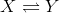The conversion ofto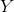releases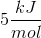of energy and has an activation energy of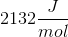.

What is the activation energy of the reverse reaction?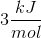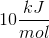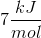Cannot be determined from the given informationExplanation:

To answer this question, we have to consider an energy diagram for an exothermic reaction. We know the forward reaction is exothermic because the question states that energy is released. We are given the activation energy of the reaction (the energy difference between the activated complex, or transition state, and the reactants) and the energy released (the energy difference between the products and the reactants). In the reverse reaction we will be going from products to reactants as follows: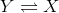This means that the activation energy for reverse reaction will be higher (has to climb a higher hill from “products” to “activated complex”). Using the given information we can deduce that the activation energy (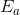) of the reverse reaction is the SUM of the activation energy of the forward reaction AND the energy released from the forward reaction.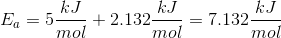The closest answer is. Note that the forward reaction is exothermic, whereas the reverse reaction is endothermic (energy is being consumed). The reverse reaction is endothermic because the reactant () has lower energy than the product ().

### Example Question #8 : Reaction Energy Profile

Which of the following is true regarding activation energy?

Increasing the temperature will decrease the activation energy

A catalyst increases the energy of the reactants

Enthalpy of a reaction has no effect on the activation energy

Activation energy determines the equilibrium constant of a reaction

Enthalpy of a reaction has no effect on the activation energy

Explanation:

Recall that the only thing that can alter the activation energy of a reaction is the addition of a catalyst. Factors such as enthalpy, entropy, temperature, and pressure don’t change the activation energy.

Catalysts decrease the activation energy and increase the rate of reaction. They have no effect on the energy of the reactants. As mentioned, temperature will not alter the activation energy; however, increasing the temperature will speed up the reaction. This occurs because adding energy in the form of heat will increase the energy of individual molecules in the reaction and will allow more molecules to overcome the energy barrier. Note, however, that temperature does not change the energy barrier (activation energy).  Recall that changing activation energy has no effect on the equilibrium of the reaction; therefore, equilibrium constant does not depend on the activation energy.

### Example Question #3 : Help With Anodes And Cathodes

Consider the following electrolytic cell: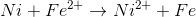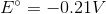What happens at the anode in the electrolytic cell?

Iron is reduced

Iron is oxidized

Nickel is oxidized

Nickel is reduced

Nickel is oxidized

Explanation:

It does not matter if the cell is galvanic or electrolytic; oxidation will always take place at the anode. This means that the nickel loses two electrons and is oxidized at the anode to generate nickel ions.Nickel ions and iron are products, and are neither oxidized nor reduced during the reaction. Iron ions are reduced at the cathode to generate the iron product.

### Example Question #1 : Galvanic (Voltai) And Elecrolytic Cells

Consider the following reaction in a galvanic cell: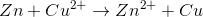Which of the following takes place at the anode?

Zinc is oxidized

Zinc is reduced

No reaction takes place at the anode

Copper is oxidized

Copper is reduced

Zinc is oxidized

Explanation:

Oxidation takes place at the anode and reduction takes place at the cathode. You can remember this with the pneumonic "An Ox, Red Cat."

In the equation, zinc loses electrons. It goes from a neutral, elemental charge to a charge of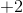. Since electrons are negative, a loss of electrons will cause an increase in charge. Because zinc loses electrons, it is oxidized. This will take place at the anode.

### Example Question #1 : Galvanic (Voltai) And Elecrolytic Cells

Toward which pole do electrons travel in a galvanic cell?

Towards the cathode

Electrons do not travel; only the protons travel

Towards the anode

Towards the negative pole

Towards the cathode

Explanation:

Reduction always occurs at the cathode, and oxidation always occurs at the anode. Since reduction is the addition of electrons, the electrons must flow toward the site of reduction.

In a galvanic cell the positive charge is on the cathode, while the negative charge is on the anode. Since a galvanic cell has a positive potential and is spontaneous, electrons freely flow down their potential gradient. The electrons, which are negatively charged, are traveling towards the cathode, which is positive charged, since opposites attract.

### Example Question #5 : Electrochemistry

Toward which pole do the electrons travel in an electrolytic cell?

Towards the cathode

The electrons do not travel; only the protons travel

Towards the anode

Towards the positive pole

Towards the cathode

Explanation:

Reduction always occurs at the cathode, and oxidation always occurs at the anode. Since reduction is the addition of electrons, electrons must travel toward the site of reduction.

In an electrolytic cell the negative charge is on the cathode, while the positive charge is on the anode. Since an electrolytic cell requires energy to perpetuate the reaction, we are pushing the electrons against their potential gradient. The electrons, which are negatively charged, are traveling towards the cathode, which is also negatively charged.

### Example Question #2 : Electrochemistry

Which of the following differences between galvanic cells and electrolytic cells is false?

Electrolytic cells have oxidation take place at the cathode

Electrolytic cells are non-spontaneous

Electrolytic cells have negative voltages

Electrolytic cells have a positive Gibb's free energy